# Harmonic series

(diff) ← Older revision | Latest revision (diff) | Newer revision → (diff)

The series of numbers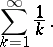Each term of the harmonic series (beginning with the second) is the harmonic mean of its two contiguous terms (hence the name harmonic series). The harmonic series is divergent (G. Leibniz, 1673), and its partial sums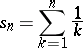increase as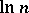(L. Euler, 1740). There exists a constant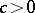, known as the Euler constant, such that, where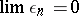. The series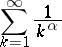is called the generalized harmonic series; it is convergent for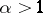and divergent for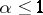.

For a proof of the expression for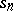see, e.g., [a1], Thm. 422. Note that the series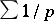extended over all prime numbersdiverges also; see, e.g., [a1], Thm. 427, for an expression of its partial sums.
Generalized harmonic series are often used to test whether a given series is convergent or divergent by estimating in terms of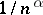the order of the terms of the given series; see Series.